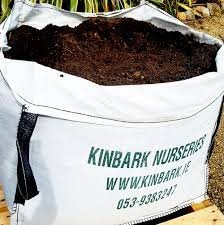# Farmer 5

Farmer Joe ordered three bags of soil last month. Each bag weighed 4 ⅖ kilograms. He used the first bag in a week. At the end of this month, there were 2 ¾ kilograms of soil left in the second bag and ⅞ kilograms of soil left in the third bag. How much soil was used this month?

u =  9 2340 = 383/40 kg

### Step-by-step explanation:Did you find an error or inaccuracy? Feel free to write us. Thank you!

Showing 1 comment:
Math student
3 1/4 + 2 5/6

Tips for related online calculators
Need help calculating sum, simplifying, or multiplying fractions? Try our fraction calculator.
Need help with mixed numbers? Try our mixed-number calculator.
Do you want to convert time units like minutes to seconds?